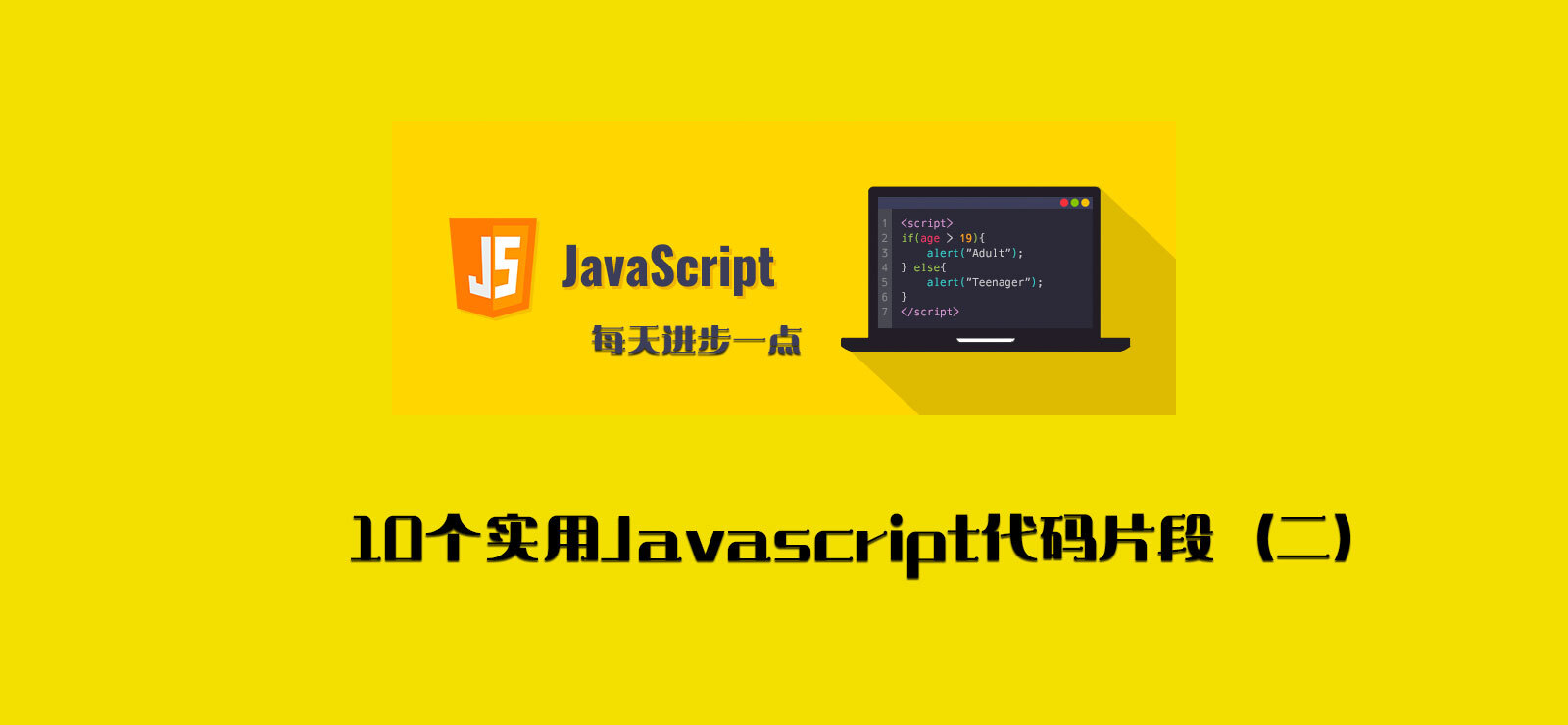# 每天学习 10 个实用 Javascript 代码片段（二）devpoint### 1. forObject

const forObject = (obj, callback) =>    Object.keys(obj).forEach((key) => callback(obj[key], key, obj));const testObj = {    title: "每天学习10个实用Javascript代码片段（一）",    author: "天行无忌",};forObject(testObj, (val, key) => {    console.log(${key}:${val});});// title:每天学习10个实用Javascript代码片段（一）// author:天行无忌

### 2. forReverse

const forReverse = (array, callback) =>    array.slice(0).reverse().forEach(callback);const testArray = [1, 2, 3, 4];forReverse(testArray, (val) => console.log(val));// 4// 3// 2// 1

### 3. flatten

const flatten = (array, depth = 1) =>    array.reduce(        (acc, current) =>            acc.concat(                depth > 1 && Array.isArray(current)                    ? flatten(current, depth - 1)                    : current            ),        []    );const arrayTest = [0, 1, 2, [[[3, 4, ]]]];console.log(flatten(arrayTest)); // [ 0, 1, 2, [ [ 3, 4, [Array] ] ] ]console.log(arrayTest.flat()); // [ 0, 1, 2, [ [ 3, 4, [Array] ] ] ]const depth = 3;console.log(flatten(arrayTest, depth)); // [ 0, 1, 2, 3, 4, [ 5 ] ]console.log(arrayTest.flat(depth)); // [ 0, 1, 2, 3, 4, [ 5 ] ]

### 4. deepFlatten

const deepFlatten = (array) =>    [].concat(        ...array.map((item) => (Array.isArray(item) ? deepFlatten(item) : item))    );const arrayTest = [0, 1, 2, [[[3, 4, ]]]];console.log(deepFlatten(arrayTest)); // [ 0, 1, 2, 3, 4, 5 ]

### 5. findKey

const findKey = (obj, fn) =>    Object.keys(obj).find((key) => fn(obj[key], key, obj));const testObj = {    hballard: { age: 36 },    sguzman: { age: 40 },    plowe: { age: 32 },};console.log(findKey(testObj, (item) => item.age > 32)); // hballard

### 6. filterDuplicate

const filterDuplicate = (arr) => [...new Set(arr)];const testArray = [1, 3, 4, 5, 5, 5, 6, 2, 9];console.log(filterDuplicate(testArray)); // [1, 3, 4, 5, 6, 2, 9]

### 7. dropWhile

const dropWhile = (array, fn) => {    while (array.length > 0 && !fn(array)) array = array.slice(1);    return array;};const testArray = [30, 29, 33, 31, 28];console.log(dropWhile(testArray, (item) => item >= 33)); // [ 33, 31, 28 ]

### 8. digitize

const digitize = (number) => [...\${number}].map((item) => parseInt(item));console.log(digitize(2021)); // [ 2, 0, 2, 1 ]

### 9 .differenceWith

const differenceWith = (array1, array2, comp) =>    array1.filter((a) => array2.findIndex((b) => comp(a, b)) === -1);const a1 = [1, 2, 3, 4];const a2 = [3, 4, 5, 6];console.log(differenceWith(a1, a2, (a, b) => a === b)); // [ 1, 2 ]

### 10. defer

const defer = (fn, ...args) => setTimeout(fn, 1, ...args);defer(console.log, "devpoint");console.log("infoq"); // 先 infoq 后 devpoint## 评论